Facilitating the Spread of Knowledge and Innovation in Professional Software Development

Write for InfoQ

### Topics

InfoQ Homepage Articles Improving the Performance of a Route Editor Using a Quadtree

# Improving the Performance of a Route Editor Using a Quadtree

### Key Takeaways

• Choosing the right data structure and algorithm for your solution is key to ensure the desired level of performance
• A quadtree is useful to partition your search space into units of correlated spatial information and reduce your problem to a set of problems of reduced size
• Do not forget that basic math can be your friend to improve your system performance
• Always measure at various steps in your algorithm refinement process to be able to gauge progress
• Prevention is better than cure, so use a quadtree from the initial stages of a project to prevent  problems later down the line

At Axmor, we develop custom IT solutions and have built our fair share of business process automation for maritime transportation. In this article, we will describe how we used a quadtree to solve a critical performance issue for an application used to plot a route on a map for river freight transport.

In any route mapping app a significant portion of processing efforts falls to the algorithm that searches and checks newly created elements for intersections with existing ones - so that you know where you are relative to where you just were or wish to go. Initially, we were doing this using a brute force approach, that is comparing elements one by one. But, after the first dozen of elements were created, the processing of intersections was time-consuming and bogged the tool down, making it slow and cumbersome to use. The solution we found was applying quadtrees to represent data in a more structured way and use a faster search algorithm.

A quadtree is a tree data structure that allows the user to partition a two-dimensional space and to quickly find the intersection of objects. Quadtrees are frequently used in fields involving a dynamic context, such as in game development or geo-indexing applications where the user needs to track a moving object, assign, or edit routes, etc.

Before going forward we would advise that prevention is better than cure, and would thus recommend to use a quadtree from the initial stages of a project to prevent problems later down the line.

## The main problem of route mapping and how to solve it

The basis for our discussion is a fairway - an optimal route for a ship traveling from point A to point B, which is represented by a set of polylines without branches (route) that connect a number of geofences (destinations). Our goal was to create a fairway editor, i.e. a tool that allows you to add new routes, delete route sections, add, or remove forks, etc.

The following picture displays a geofence, represented through the orange-filled shape, and various routes close to it.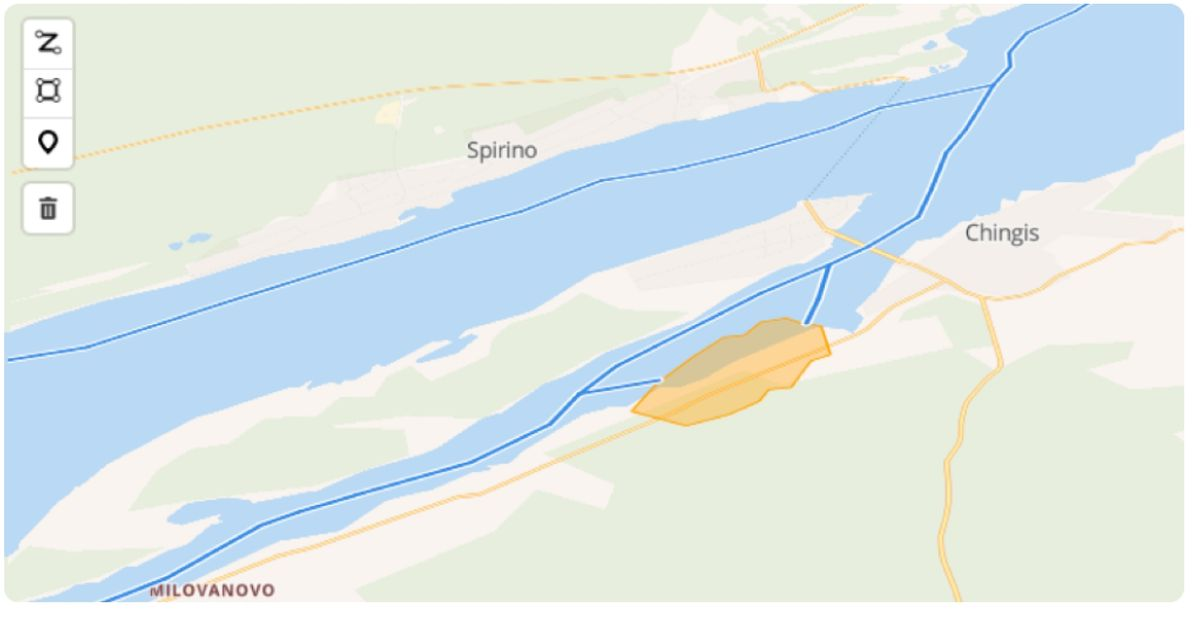In addition to support the basic operations to create the fairway, we also need to track the areas adjacent to any geofence, and to dynamically change and display their current state on the map.

To solve this problem, we break down the initial model into a simpler one, more useful for performing atomic user operations. So, we divide the set of polylines into line segments, resulting in a set of line segments, points, and geofences. Upon completion of the editing process, we merge short line segments to form long polylines connecting the so-called "special" points: boundary points, branch points, and points adjacent to geofences.

When editing the model (i.e. adding or deleting a point or line segment, etc.), we need to recalculate the intersections of line segments, to determine whether any geofences are close to any newly added point or line segment, to update the properties of the edited points and of the entire model, and then to store the information about the areas that have been added, updated, or changed.

When there are only a few points on the map, all these calculations take a fraction of a second. However, as more of the fairway is plotted, performance gradually arises as an issue. In our case, the initial data loading at some point began to take up to 20-40 seconds, and a simple user action, such as connecting 2 points, took about 8 seconds. The response time of the system became unacceptably slow, rendering the solution unusable for even the most patient of our users. The need for optimization was obvious.

The basic idea we came up with was to limit the area of recalculation. For example, when adding a new point it is not necessary to go through all the geofences to find those that are close to it. Instead, it is enough to cycle through the geofences located in close proximity to the point in question, thereby reducing beforehand the list of objects on which "heavier" calculations will be applied.

The algorithm we used to restrict the recalculation area and speed up the calculations has two steps: building a quadtree followed with a projection intersection check, as we describe below.

The first step is to apply a quadtree to the model currently in use.

The quadtree is populated with the elements of a model (points, line segments, and geofences) and then it is used to search for objects in a limited area. For example, a point can give us the location of nearby geofences and line segments. A line segment can point us to other nearby line segments, etc.

Even at this first stage, for a fairway model consisting of 2500 points, 2500 line segments,  and 180 geofences, the number of objects to search has significantly decreased (from the initial 2500 to 2-40, depending on the type of object).

At the second stage, we exclude from the list of objects we got from step one those which fail to satisfy the necessary condition for intersection, i.e., the fact that if two objects on the plane intersect, then their projections on the coordinate axes also intersect. We included this condition as an additional quick check for intersections. After these two stages, the editor can perform basic calculations and manipulations with the few remaining objects.

Thanks to the two steps detailed above, we were able to significantly speed up calculations and reduce response time to user actions. As a result, all calculations for data generation are now completed in under a minute, and user actions are processed in less than 200 milliseconds.

## The inner workings of the system

We now have a fairly general understanding of the problem and its solution. The remaining section of this article will delve into more detail in the inner workings of our system and will provide a thorough explanation of the way we used quadtrees to improve performance.

### Available user actions

In simple terms, a fairway can be modelled as a set of line segments and points (the ends of the line segments).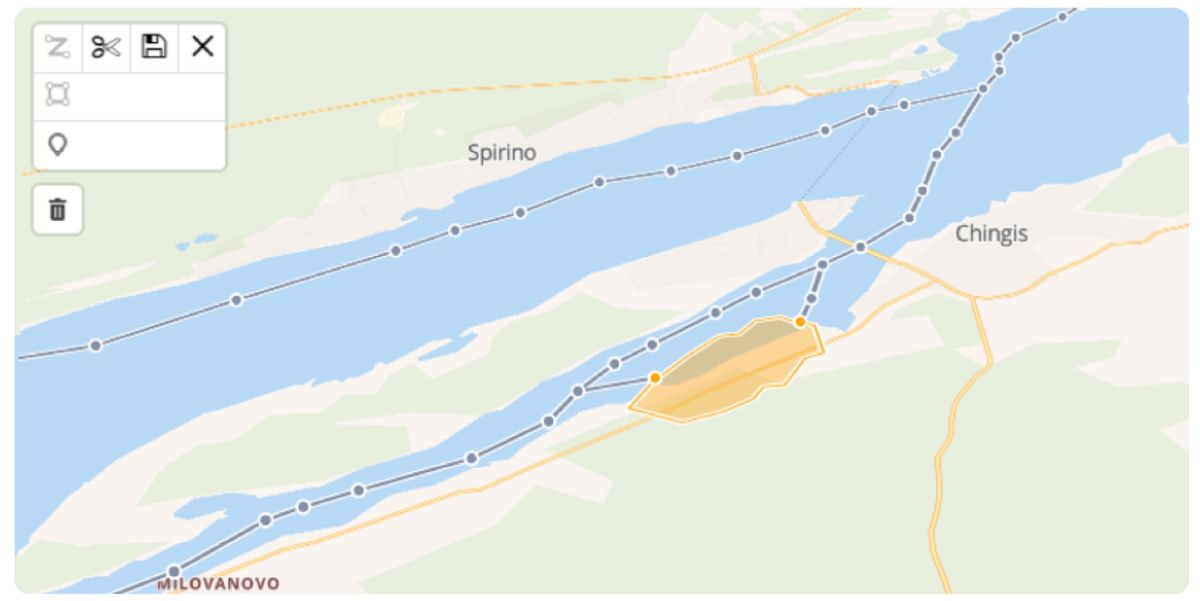To build a fairway model, the user can carry through a number of possible operations.If the user clicks on an empty space, a new active point is created.

If the user clicks inside a line segment (except for the line ends), that segment is divided in two.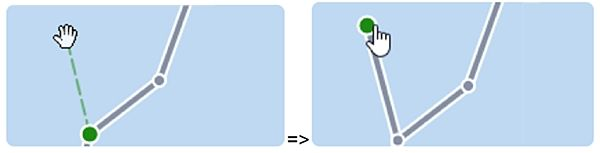If a user clicks on an existing point, it becomes active.

If a new point is created when another point is active, the two points are connected by a new line segment. Moreover, all intersections of the new line segment with existing ones  create new points.

• Deleting a pointThe user can delete the active point. If the deleted  point connects 2 line segments, only one line segment will remain. Instead, if the point belongs to one line segment only, the whole line segment is deleted along with it.

• Deleting a line segmentThe user can select a line segment and delete it. If the line segment was part of a polyline, the polyline is broken.

• Moving a point and adjacent segments using drag-and-drop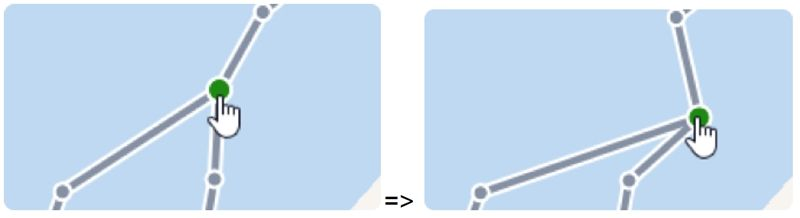The user can drag and drop the active point, thus making all line segments adjacent to it change their position. If new intersections between line segments are created by this operation, the editor searches all of them and adds a new point for each.

If a point overlaps with another point, the two points are combined to form a single one. Likewise, if one segment is overlapped with another, the two segments are joined.

### Types of points

As explained, the model of a fairway consists of points and line segments connecting them.

A point can be alone, i.e. not connected to other points, or it can be connected to other points through line segments.

Points are classified by the number of line segments they connect. The operations that can be performed on a point depend on the point’s type. We can have the following types of points.

Single point

A single point does not belong to any line segment. On the map, a single point is always represented as an active point that has not yet been connected to another point by a line. Available operations: delete, move.

End point

An end point belongs to precisely one line segment. Available operations: delete, move. If the user deletes an end point, the segment adjacent to it is deleted, too.

Middle point

A middle point connects two line segments. Available operations: delete, move. Upon deletion of a middle point, the two segments it connects are merged into one.

Branch point

A branch point belongs to  three or more line segments. Available operations: move. The option to delete a point of this type is not available, since the result of such an action would be unclear.

### System calculations

The system stores data in maximally unbranched routes from knot to knot (usually a major destination point). In order to edit a route, the system first needs to convert it into simple elements like line segments and points. Then, it performs primary calculations with the obtained elements, as well as the previously known geofences.

User actions, such as adding, deleting, or moving points (and thus line segments), result in the need to recalculate the model. For example, if any existing point or line segment lays close to a new point, we want to merge them together; if an existing line segment intersects with a new or modified line segment, we want to split them and add a connecting point; Similarly, we need to calculate the intersection of new and modified points with any geofences; as well as to find and remove duplicate points and line segments.

When the user is done editing, the editor combines the line segments back into maximally unbranched routes.

Features of calculations

A special adjustment had to be made in the calculations for close points. It is nearly impossible to click on the same spot on the map twice and get a point with the same coordinates. That is why when two points are "sufficiently close", the editor merges them into a single point. The same is true for intersections and line segments.

Given all this, it should be apparent that the primary and most frequent operation on our model is comparing new and modified model elements with existing ones, to find those that are located close to each other. To restrict the multitude of enumerated objects to be searched and speed up the search within the restricted set, we selected a quadtree.

The quadtree is used to gain quick access to objects located in a defined spatial area. Each quadtree leaf is recursively divided into 4 quadrants, then into sub-quadrants, and so on. Hence, we have a system of nested spatial regions (in simple terms, rectangles or squares), with each quadtree leaf corresponding to a specific spatial area. Each node of the tree either has 4 children or none

Quadtree nodes may contain model objects such as line segments and points, which represent fairways,  and polygons, which are used to represent geofences.

The following code snippet shows how a quadtree can be represented:

class QuadTree {

maxItems: number; // MaxItems is a constant, and represents the maximum number of items that can be stored in a single node
boundary: AABB; // Bounding box aligned along the coordinate axes shows the tree boundaries
level: number;  // The depth or level of the tree
points; // Points
segments; // Segments
polygons; // Geofences

// Pointers to child tree nodes:
}

To add a line segment, the system recursively searches for the smallest quadtree leaf that contains it. This is a leaf of the tree that contains the new line segment, with the additional condition that none of its children contains the entire line segment.

This implies that, starting from the root node of the tree, the system visits each child leaf to check if it contains the specified line segment. If it does, then the system recursively visits its children to check whether one contains the line segment. When none of the children contain this line segment, then the line segment is inserted into the current leaf.

The above algorithm is sketched in the following code snippet.

public insert(segment): boolean {

// Returns false if the objects do not belong to the tree
if (!this.boundary.contains(segment))
return false;

// Next, divide the area and check if we can add a segment to any child node
if (this.northWest == null) {

// Next, we create sub-quadrants for the current quadrant/node, if they are not there yet, and check if we can add the segment to any of them
}

if (this.northWest.insert(segment)) return true;
if (this.northEast.insert(segment)) return true;
if (this.southWest.insert(segment)) return true;
if (this.southEast.insert(segment)) return true;

// Failed to insert into any child element - insert into current node
this.segments.push(segment);

//… initialize auxiliary properties of the object

return true;

}

To add a polygon to the quadtree, the system recursively searches for the smallest quadtree leaf containing a polygon.

Taking into account the fact that we are searching for non-precise intersections, the system will search for a quadtree leaf in which the polygon is not adjoining the leaf borders, but is at some distance delta from them.

In other words, we are searching for a quadtree leaf that entirely contains the polygon including a margin not less than the specified delta, while none of its children satisfies this condition.

The algorithm is the same as when inserting a line segment into the quadtree.

With regards to points, the process is the same as when inserting polygons. We search for a quadtree leaf that meets the following conditions: it contains a point and the point is located at a distance not less than delta from the leaf boundaries.

At the same time, since a point-plus-delta is a very small object in relation to any geographical area designated as a first level quadtree leaf, it runs a risk of being assigned to an infinitely small leaf at the deepest level of division. To limit the system’s strive for accuracy and balance tree depth with the number of elements within each leaf, we designate the initial number of divisions (MIN_LEVEL) and maximum allowed number of divisions (MAX_LEVEL) to prevent the system from going deeper than what initially specified  in case the number of elements per leaf exceeds what we consider optimal (NODE_CAPACITY). Thus, the distribution of points across the leaves follows these rules:

• The number of points in a tree leaf should not exceed the specified NODE_CAPACITY threshold. In our current solution, a quadtree leaf can hold a maximum of four points. When there are more elements within a leaf, the leaf is divided and a next level is created.
• The initial division level for a new point is described by MIN_LEVEL. Points are inserted into the tree starting at this level. We used the value  MIN_LEVEL = 12. We chose the number experimentally, taking into account general considerations. Were it not for this restriction, points would first be added at level one, and when this is filled up - at level 2, and so on. This would make most of them end up in the first three levels and therefore show up in almost all of the query results. Level 12 gives us a good-enough precision while not overcrowding the tree with leaves.
• The depth of the tree is bound atby MAX_LEVEL. We used the value  MAX_LEVEL = 100. This limitation had a large margin, and was never actually reached. This condition may contradict the first condition, but it is considered to be of higher priority.

Thus, the condition for inserting a point into the tree looks like this:

If (this.level == MAX_LEVEL ||
(this.level > MIN_LEVEL && this.points.length < NODE_CAPACITY))
{
//insert point to this leaf
}

### Finding intersections in a given area

Now we come to the main source of potential performance usage, namely the search. All in all, we have tried to apply four algorithms when dealing with continuous recalculations of relative positions and intersections of objects and geozones.

1. Brute force + definitive intersection, or simple search algorithm, that compares all couples of elements one by one and checks whether they intersect by applying calculations to their coordinates.
2. Quadtree + definitive intersection - structuring the data prior to search with the help of a quadtree, where each element is assigned to a tree leaf, comparing only neighbouring elements according to the tree, and then applying calculations only to the resulting, much reduced set of elements.
3. Brute force + intersection of projections + definitive intersection - the algorithm checks couples of elements one by one to see if projections of coordinates of two objects intersect, and therefore the objects themselves may intersect. If they do not, the object is excluded from the list. If they do, the object is included in further calculations. The reason why this method is effective is projection-based comparison is much faster than checking for intersection using coordinates, therefore this helps to eliminate non-intersecting objects quickly.
4. Quadtree + intersection of projections + definitive intersection. A combination of 2 and 3, where the list is first limited by neighbouring objects, then checked for intersection of projections, and finally the definitive intersection is found by coordinates.

We settled on the fourth method as the fastest. Below we explain how our resulting algorithm works and provide performance measurements from the tests we have run for comparison.

To find objects that intersect one another (line segments, points, geofences, or combinations of them), or objects located close to each other, we need to intersect the quadtree leaves in which the objects are located.

After we construct the quadtree and assign each object to a quadtree leaf, the set of objects potentially intersecting the given object is easy to find in the following way: for a tree leaf that contains the given object (point, line segment or polygon), we take all tree leaves that intersect with it. Thus, we take the leaf itself in addition to all its parent elements up to the root of the tree and, recursively, all the children of the given leaf and all of its descendants. Having obtained this set of leaves, we select all elements of the required type (point, line segment or polygon) with which we are trying to find the intersection.

### Applying the necessary condition for the intersection of projections

The initial levels of the tree include both large objects and very small ones located on the border of the quadrants. Objects that are located on quadrant boundaries, as well as large objects, will be returned in most queries. Therefore, the first request returns several dozen "extra" elements located at a sufficient distance from each other, even for a relatively evenly distributed model without large elements.

In order to discard those small objects that are located at the boundaries of quadrants and fall into almost all queries, we use an additional necessary condition for the intersection of projections. When configuring points and segments for the fairway, the result is an average of 2-8 objects, with which we can make more accurate calculations.

As mentioned, the necessary condition for intersection follows from the fact that if two objects belonging to the same plane intersect, then their projections on the coordinate axes also intersect. This is true for lines, points and polygons.

This means that if the projections of two objects do not intersect, then the objects do not intersect.

Thus, to search for intersections of points, lines, polygons and their combinations, we need to check whether their projections intersect. If they do not intersect, we can exclude the object from the query result.

Since the solution to our problem does not require an exact intersection, we apply the same principle as before: we look for the intersection of projections enlarged from both sides by delta.

Expressing those conditions in terms of code is left as an exercise to the reader.

### Performance measurements

Our web application was written in JavaScript using Angular 6 + TypeScript.

We used Mapbox GL JS to display maps and navigational elements; mapbox-gl-draw to interact with the map and edit the navigational elements; Turf.js, an auxiliary JavaScript library for working with geo objects, to calculate intersections.

At the time of taking performance measures, we used the fairway that had been already plotted, and consisted of approximately 2596 line segments and 2549 points.

Despite the fact that the route was plotted fairly evenly along the river (i.e. there was no cluttering of elements), after applying only the quadrant tree algorithm, the number of line segments in a given area turned out to be larger than expected by a factor of ten, as, on the border of two tree leaves many small objects will be assigned to their parent and will be included in most queries.

The three tables and the diagram below show execution times for input data of 725, 1291 and 2596 segments using the four approaches described above. The figures are approximate, nevertheless they serve to illustrate the effectiveness of the algorithms.

Here we provide the average data loading time and the average time required to perform one atomic operation, such as adding/moving/deleting a point or a line segment.

In the tables below, in addition to the average times, we show the average number of line segments  and the average number of points returned for a given line segment after applying the algorithm.

1. Input data - 725 segments, 770 points, 146 geofences. Maximum leaf level (depth) of the tree = 18, maximum number of segments in the leaf = 5, maximum number of points in the leaf = 8.

 Algorithms used Average number of segments Average number of points Average time to load data Average time to complete an atomic operation simple search 725 770 3913 ms 630 ms quadtree 15-20 2-8 349 ms 40-60 ms intersection of projections 3 0-2 2100 ms 920 ms quadtree and intersection of projections 3 0-2 240 - 300 ms 40-60 ms

2. Input data - 1291 segments, 1336 points, 146 geofences. Maximum leaf level (depth) of a tree = 19, maximum number of segments in a leaf = 6, maximum number of points in a leaf = 8.

 Algorithms used Average number of segments Average number of points Average time to load data Average time to complete an atomic operation simple search 1291 1336 8866 ms 1900 ms quadtree 10-20 2-8 508 ms 45-80 ms intersection of projections 2-4 0-2 5400 ms 2750 ms quadtree and intersection of projections 2-4 0-2 380 - 500 ms 60-80 ms

3. Input data - 2596 segments, 2549 points, 146 geofences. Maximum leaf level (depth) of a tree = 19, maximum number of segments in a leaf = 8, maximum number of points in a leaf = 10.

 Algorithms used Average number of segments Average number of points Average time to load data Average time to complete an atomic operation simple search 2596 2549 1175 ms 7000-8000 ms quadtree 20-30 5-20 349 ms 100-200 ms intersection of projections 2-8 0-2 18000-20000 ms 10000-11000 ms quadtree and intersection of projections 2-8 0-2 800 - 900 ms 100-200 ms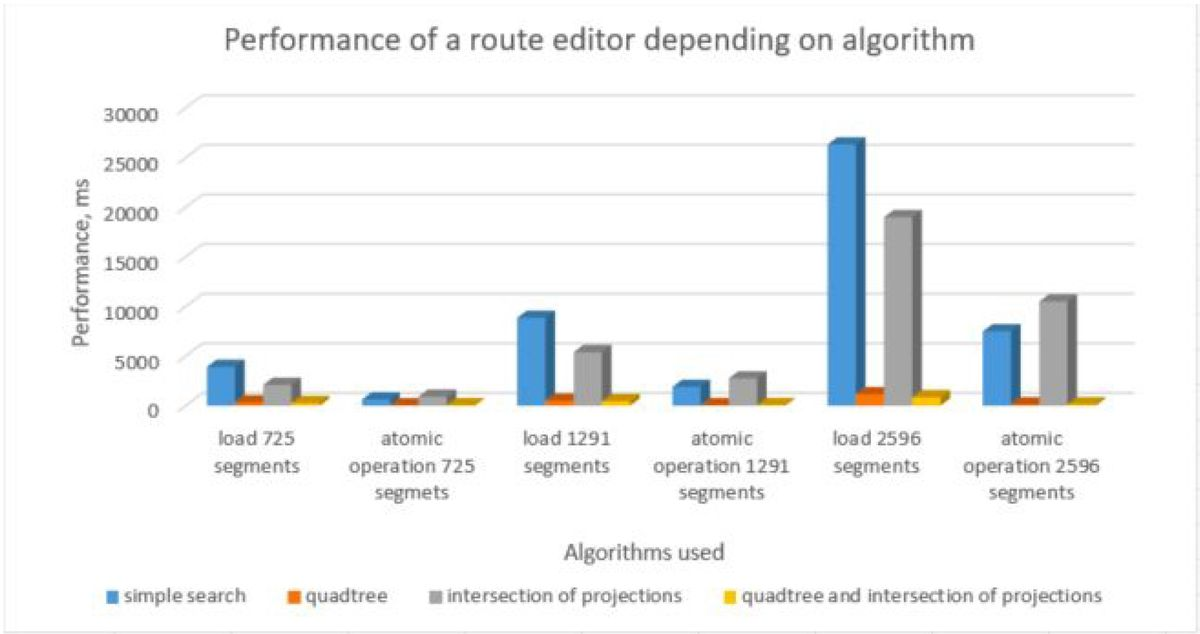## Conclusions

As we have shown here, the quadtree is both simple to implement and efficient to use, greatly reducing the number of objects to iterate over. The tree is quickly constructed and is used to find objects in a given area with a high degree of efficiency. Even if the system performance currently falls within acceptable limits, it may be worth applying a quadtree. This algorithm has been shown to be appropriate for tasks such as the construction of a fairway, when the model is distributed in space.

Using a quadtree and enforcing the necessary condition for intersection of projections, significantly reduced the time for loading, editing, and updating application data.Nina Fursova is a full-stack developer with over 12 years of experience and passion for computational maths. For the past 6 years she's been specializing in web applications on JavaScript and Angular and is a software engineer at Axmor.

Style

## Hello stranger!

You need to Register an InfoQ account or or login to post comments. But there's so much more behind being registered.

Get the most out of the InfoQ experience.

Allowed html: a,b,br,blockquote,i,li,pre,u,ul,p